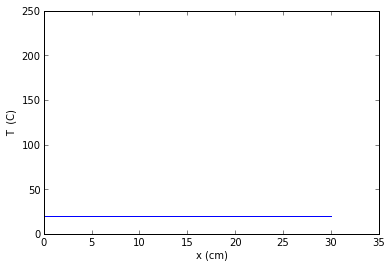# Chapter 4: Unsteady State heat Conduction¶

### Example 4.1 Page No.190¶

In :
k=12.0          #  thermal conductivity in BTU/(hr.ft.degree Rankine)
c=0.1         # specific heat in BTU/(lbm.degree Rankine)
D=0.025/12.0    # diameter in ft
density=525.0   # density in lbm/cu.ft
hc=80         # convective coefficient in BTU/(hr. sq.ft. degree Rankine)
T_i=65.0        # intial temperature in degree fahrenheit
T_inf=140.0      # fluid temperature in degree fahrenheit
As=3.14*D**2    # surface area in sq.ft
Vs=3.14*D**(0.5)  # volume in cu.ft

import math
reciprocal_timeconstant=(hc*6)/(density*D*c)
T=139
t=math.log((T-T_inf)/(T_i-T_inf))/(-reciprocal_timeconstant)

print"The response time of the junction is %.1f s",round(t*3600,2),"s"

The response time of the junction is %.1f s 3.54 s


### Example 4.2 Page No. 193¶

In :
k=236.0         # thermal conductivity in W/(m.K)
Cp=896.0        # specific heat in J/(kg.K)
sp_gr=2.702     # specific gravity
density=2702.0  # density in kg/cu.m
D=0.05          # Diameter in m
L=0.60          # length in m
hc=550.0        # unit surface conductance between the metal and the bath in W/(K.sq.m)

import math
Vs=(math.pi*D**2*L)/4.0                # Volume in cu.m
As=(2*math.pi*D**2/4.0)+(math.pi*D*L)  # surface area in sq.m
import math
Bi=(hc*Vs)/(k*As)     # Biot Number
T_i=50.0         # initial temperature in degree celsius
T_inf=2.0        # temperature of ice water bath in degree celsius
t=60.0           # time=1 minute=60 s
As_=0.102        #approx value taken in book for calculating T and Q
T=T_inf+(T_i-T_inf)*math.exp(-(hc*As_*t)/(density*Vs*Cp))
Q=density*Vs*Cp*(T_inf-T_i)*(1-math.exp(-(hc*As_*t)/(density*Vs*Cp)))

print"(a)The temperature of aluminium is",round(T,1),"C"
print"(b)The cumulative heat transferred is ",round(-Q/1000,1),"KJ"

(a)The temperature of aluminium is 16.7 C
(b)The cumulative heat transferred is  94.8 KJ


### Example 4.3 Page No. 200¶

In :
hc=30
L=0.24
k=1.25           #Conductivity
c=890
rou=550
Fo=0.4           #Fourier no

Bi=hc*L/k
alpha=k/(rou*c)
Tc=150
T_inf=600
T_i=50
t=(L**2*Fo)/(alpha)
TC1=0.82       #Centreline temprature
T=0.71*(T_i-T_inf)*TC1
x=0.4*L
Ti=149
To=492
print"Time required to reach temprature 150 is ",round(t/3600,2),"hr"

Time required to reach temprature 150 is  2.51 hr


### Example 4.4 Page No. 204¶

In :
hc=6         #Surface Conductance
D=0.105       #Orange Diameter
k=0.431      #Thermal conductivity
c=2000       #Specific heat of orange
rou=998      #Density
Fo=1.05        #Fourier no.

import math
Vs=math.pi*D**3/6
As=math.pi*D**2
Bi=hc*Vs/(k*As)
Bi_=hc*(D/2)/(k)
alpha=k/(rou*c)
Tc=20
T_inf=23
T_i=4
t=(Fo*(D/2.0)**2)/alpha
a=Bi_**2*Fo
Q=0.7*rou*c*(math.pi/6.0*(Fo**3))*(T_i-T_inf)

print"The time required is ",round(t/3600,2),"hr"
print"The heat transfered is",round(Q/1000,2),"kj"

The time required is  3.72 hr
The heat transfered is -16090.84 kj


### Example 4.5 Page No.208¶

In :

D=0.105          #diameter
k=0.3            #Thermal conductivity
c=0.41          #Specific heat
sp_gr=2.1         ##Specific gravity
rou_water=62.4   #Density
alpha=k/(sp_gr*rou_water*c)
t=3*30*24

T_inf=10
Ts=10
T=32
T_i=70
dimensionless_temp=(T-T_i)/(T_inf-T_i)
variable_fig4_12=0.38   #The value of x/(2*(alpha*t)**0.5) from figure 4.12
x=2*math.sqrt(alpha*t)*variable_fig4_12

print"The depth of the freeze line in soil is  ft",round(x,2),"ft"

The depth of the freeze line in soil is  ft 2.64 ft


### Example 4.6 Page No.211¶

In :
k_al=236
p_al=2.7*1000
c_al=896
k_oak=0.19
p_oak=0.705*1000
c_oak=2390

import math
math.sqrt_kpc_al=math.sqrt(k_al*p_al*c_al)
kpc_R=4
T_Li=20
T_Ri=37.3
T_al=(T_Li*(math.sqrt_kpc_al)+T_Ri*math.sqrt(kpc_R))/(math.sqrt_kpc_al+math.sqrt(kpc_R))
math.sqrt_kpc_oak=math.sqrt(k_oak*p_oak*c_oak)
T_oak=(T_Li*(math.sqrt_kpc_oak)+T_Ri*math.sqrt(kpc_R))/(math.sqrt_kpc_oak+math.sqrt(kpc_R))

print"The temperature of aluminium is felt as ",round(T_al,2),"C"
print"The temperature of oak is felt as %.1f degree celsius",round(T_oak,1),"C"
print"So oak will feel warmer to the touch than will the aluminium"

The temperature of aluminium is felt as  20.0 C
The temperature of oak is felt as %.1f degree celsius 20.1 C
So oak will feel warmer to the touch than will the aluminium


### Example 4.7 Page No.215¶

In :
rou=62.46
cp=0.9988
k=0.345
alpha=k/(rou*cp)
D=2.5/12.0
L=4.75/12.0

Vs=math.pi*D**2*L/4
As=(math.pi*D*L)+(math.pi*D**2)/2
Lc=Vs/As
hc=1.7
Bi=hc*Lc/k
t=4

Fo_cylinder=alpha*t/(D/2)**2
Bi_cylinder=hc*(D/2)/k
reciprocal_Bi_cylinder=1/Bi_cylinder
dim_T_cylinder=0.175 #The value of dimensionless temperature of cylinder from figure 4.7a at corresponding values of Fo and 1/Bi

Fo_plate=alpha*t/(L/2)**2
Bi_plate=hc*L/(2*k)
reciprocal_Bi_plate=1/Bi_plate
dim_T_plate=0.55 #The value of dimensionless temperature of infinite plate from figure 4.7a at corresponding values of Fo and 1/Bi

dim_T_shortcylinder=dim_T_cylinder*dim_T_plate
T_inf=30
T_i=72
Tc=dim_T_shortcylinder*(T_i-T_inf)+T_inf
dim_Tw_cylinder=0.77 #The dimensionless temperature from figure 4.7b corresponding to the value of 1/Bi and r/R=1
dim_Tw_plate=0.65 #The dimensionless temperature from figure 4.6b corresponding to the value of 1/Bi and x/L=1
dim_Tw_shortcylinder=dim_Tw_cylinder*dim_Tw_plate
Tw=dim_Tw_shortcylinder*(Tc-T_inf)+T_inf

print"The temperature at centre of can is %.1f degree celsius",round(Tc,0),"F"
print"The bear temperature near the metal of the can is",round(Tw,0),"F"

The temperature at centre of can is %.1f degree celsius 34.0 F
The bear temperature near the metal of the can is 32.0 F


### Example 4.8 Page No. 219¶

In :
rou=7817        #Density
c=461          #Specific heat
k=14.4         #Thermal conductivity
alpha=.387e-5
L1=0.03
L2=0.03
L3=0.04
x=0.04
T_i=95          #Internal temprature
T_inf=17         #Temprature at infinity

L=L1/2
hc=50
reciprocal_Bi_plate=k/(hc*L)
Tinf=0.085       #Temprature distribution for infinite plate
Tsi=0.225             #Temprature distribution for semi infinite plate
T=(Tinf**2)*(1-Tsi)*(T_i-T_inf)+T_inf
t=350

print"At a time 3000s The temprature is ",round(T,1),"C"
print"From the table The time requires to reach tempratue 50C is ",t,"s"

At a time 3000s The temprature is  17.4 C
From the table The time requires to reach tempratue 50C is  350 s


### Example 4.9 Page No.226¶

In [ ]:
%matplotlib inline

In :
rou=0.5*1000
cp=837
k=0.128
alpha=0.049e-5
Ti=20      #Initial temprature
dt=0.5*(0.05)**2/alpha

p=0
T0=200
m=1
T11=(Ti+T0)/2.0
m=2
T21=(Ti+Ti)/2.0
m=3
T31=(Ti+Ti)/2.0
m=4
T41=(Ti+Ti)/2.0
m=5
T51=(Ti+Ti)/2.0
m=6
T61=(Ti+Ti)/2.0

p=1
m=1
T12=(Ti+T0)/2.0
m=2
T22=(Ti+T12)/2.0
m=3
T32=(Ti+T21)/2.0
m=4
T42=(Ti+T31)/2.0
m=5
T52=(Ti+T41)/2.0
m=6
T62=(Ti+T51)/2.0
t=4.97
print"The time that will pass before the heat added",t,"hr"

import matplotlib.pyplot as plt
fig = plt.figure()

x1=[0,30]
T1=[20,20]

xlabel("x (cm)")
ylabel("T  (C)")
plt.xlim((0,35))
plt.ylim((0,250))

a1=plot(x1,T1)

The time that will pass before the heat added 4.97 hr### Example 4.10 Page No. 231¶

In :
rou=7.817*62.4      #density
c=0.110
k=8.32
alpha=0.417e-4
dx=1/12.0
Fo=1

dt=Fo*dx**2/alpha
n=8       #Enter the number of time intervals from Saulev plot
time=n*dt

print"The required time is  hr",round(time/3600,2),"hr"

The required time is  hr 0.37 hr